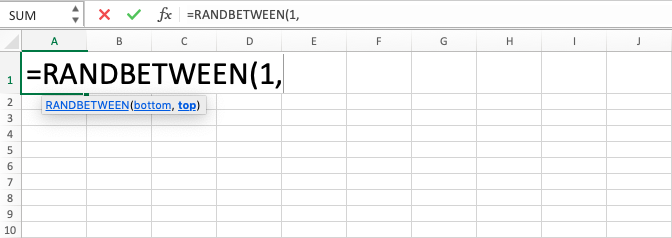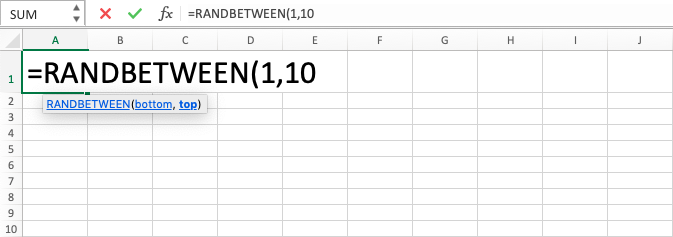RANDBETWEEN in Excel - Compute Expert

# RANDBETWEEN in Excel

In this tutorial, you will learn the RANDBETWEEN excel formula. RANDBETWEEN in excel can help you to get a random number with the lowest and the highest limit of it can be determined by you.

## Why do We Need RANDBETWEEN Excel Formula?

When doing various data processing in a spreadsheet, sometimes we need to get a digit randomly for a particular purpose. Things like task assignment or group determination sometimes need randomization so it can be done fairly and things like this can be achieved if we can find an objective method to do it.

As the illustration of its application needs in work or business, for example, imagine that we need to do group division for a company gathering activity or when we need to assign products to be sold by sales in our company with the assumption that the characteristic and detail of each product is similar one another or probably the determination of a winner of the prize from the promotion activity done for a company’s product. When we need to get the results of those fairly so there will be no pretension, it might be quite hard to say that the determination process is done objectively if we do it manually.

In these cases, it is better if the process can be done automatically to get random numbers that answer all those needs. For that, you can use RANDBETWEEN in excel to do it.

This RANDBETWEEN excel formula will help you to get numeric data automatically from the lowest and highest limit that you want. It is very useful to use if there is a need for an objective determination process which involves number randomization for the data in a spreadsheet.

## What is RANDBETWEEN in Excel?

RANDBETWEEN in excel is a formula with the function to get a random digit from a particular lowest and highest limits. RANDBETWEEN excel formula can only give a whole number result. It cannot have a decimal for a result.

RANDBETWEEN excel formula will always change its result when the worksheet where it resides is opened or there is a change in the worksheet. If you want the result to be static, then copy the cell where you put the RANDBETWEEN in excel and do Paste Special Values Only.

Generally, the input in the this formula can be described as follows:

=RANDBETWEEN(bottom, top)

Notes:
• bottom = the lowest limit
• top = the highest limit

## How Can We Use RANDBETWEEN Excel Formula?

The following will explain in detail how to write RANDBETWEEN in excel. This formula needs two required inputs: the lowest and highest limits of the random digit. The two limits are inclusive in the process so it can give the result from the digit of one of the two limits.

## Using RANDBETWEEN in Excel

1. Type equal sign ( = ) in the cell where you want to put the result in2. Type RANDBETWEEN (can be with large and small letters) and open bracket sign after =3. Input the lowest limit or cell coordinate where it is in after open bracket sign then type a comma sign ( , )4. Input the highest limit or cell coordinate where it is in5. Type close bracket sign6. Type Enter
7. The process is done!## Exercise

After you have known about the detailed steps for writing the RANDBETWEEN excel formula, now you can do the exercise to deepen your understanding!

### Questions

1. In each cell of column 5, do the randomization process with the lowest limit on column 1 and the highest limit on column 2 in each corresponding row!
2. In each cell of column 6, do the randomization process with the lowest limit on column 1 and the highest limit on column 3 in each corresponding row!
3. In each cell of column 7, do the randomization process with the lowest limit on column 2 and the highest limit on column 4 in each corresponding row!

• If you need to get a decimal randomization result, then you can divide the result of this formula with the multiplication factor of 10 as needed
• If you need to get a random number based on certain multiplication factor, then you just need to multiply the result of RANDBETWEEN excel formula with the multiplication factor that you need

Get updated excel info from Compute Expert by registering your email. It's free!